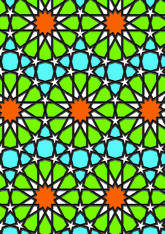# Sultan Barsbay Funerary Complexdata180/EGY1030

## Geometry

• The symmetry group of the tiling is 2*22 (cmm).
• All the internal angles of the constituent polygons are a multiple of 15°.
• Contains one regular three-pointed star polygon with vertex angle of 90°.
• Contains one regular four-pointed star polygon with vertex angle of 120°.
• Contains one regular 12-pointed star polygon with vertex angle of 60°.
• There are four non-regular reflective tiles (including one kite) and one reflective pair.
• The tiling satisfies the interlace condition and has no finite interlace and two infinite interlaces with straight cross-overs.
• The tiling is edge-to-edge.
• As drawn, contains about 449 polygons.

## References

Publications referenced: